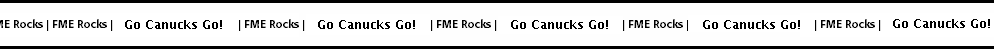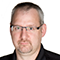Hi FME’ers,
Another short post – this time inspired by a question on the GIS StackExchange group.

The question can be found here, and was:

I am working on a series of footpath routes and have been asked if it possible to calculate the total ascent that a walker would have climbed if they travelled along each route? I know that it is simply not a case of max-min because the footpath is not just one single climb but has many stages where the path ascends and descends.

Presently, I have the data as a series of polylines and an underlying dtm.

Sounds like another case for the FME Superhero!Method
This question was quite timely because my previous post was about how to turn a 3D transit line feature into a cross section (like below). So, having done that – or, at least, using the same data – can I calculate what the actual ascent is?Well, yes I can. It took about ten minutes to create a solution. Here’s the workspace (click to enlarge):If you think you recognize the first part, then you could well be correct. It’s the same as I used in the previous post to actually create the data by draping the line over the DEM.

Here’s the key processing part:The steps are:

1. Chop the transit polyline into a series of two point lines
2. Extract the Z coordinates for the start/end of each two point line
3. Calculate the difference between Z1 and Z2
4. Sum the differences with the StatisticsCalculator

The bit I’m proudest of is the ExpressionEvaluator and how it calculates the offset. That’s because it has a built in check for where Z2 < Z1 (ie that line descends, not ascends).

The expression is:

`@Value(_z1)<@Value(_z2) ? @Value(_z2)-@Value(_z1) : 0`

It uses a technique you can find described here on fmepedia.

And, finally, if there were multiple routes/features I wanted to calculate separately, I would just use a Group-By in the StatisticsCalculator to group by route ID.Results
So, if you merely subtract the start elevation (151.471) from the end elevation (289.637) you get the result 138.166 feet of ascent. But – as the questioner notes on StackExchange – it is not a simple case of max-min because the line has sections where it ascends and descends.

The correct answer, according to the workspace, is: 266.887No, sir. Don’t thank us. We’re all part of the same team. Good night.3D About FME Coordinates Data Transformation DEM FME Desktop GIS Raster StatisticsMark Ireland

Mark, aka iMark, is the FME Evangelist (est. 2004) and has a passion for FME Training. He likes being able to help people understand and use technology in new and interesting ways. One of his other passions is football (aka. Soccer). He likes both technology and soccer so much that he wrote an article about the two together! Who would’ve thought? (Answer: iMark)